## 7.3 Data analysis

Before starting to build any model it is good practice to analyze the data. Data analysis is done in two inter-linked steps, exploratory and quantitative data analysis and therefore can be viewed together.

• Exploratory data analysis

• Find correlations or mutial depence
• Quantiative analysis
• Check distribution
• Long tail => log of variable
• Why data analysis?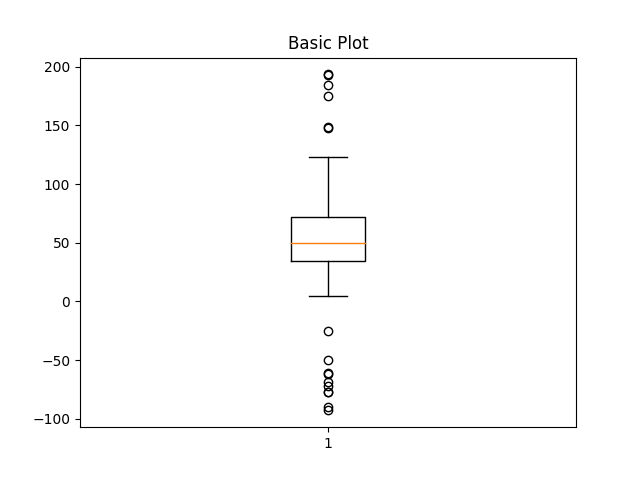• Understanding characteristic and distribution of response
• histogram
• box plot
• Uncover relationships between predictors and response
• scatter plots
• pairwise correlation plot among predictors
• projection of high-dimensional predictors into lower dimensional space
• heat maps across predictors

The process of exploratory and quantitative data analysis is described in detail in the following example.

### 7.3.1 Example for exploratory and quantitative data analysis

This example is from the online book “Feature Engineering and Selection: A Practical Approach for Predictive Models”

#### 7.3.1.1 Visualization for numeric data

In this example the data set on ridership on the Chicago Transit Authority (CTA) “L” train system http://bit.ly/FES-Chicago is used to predict the ridership in order to optimize operation of the train system.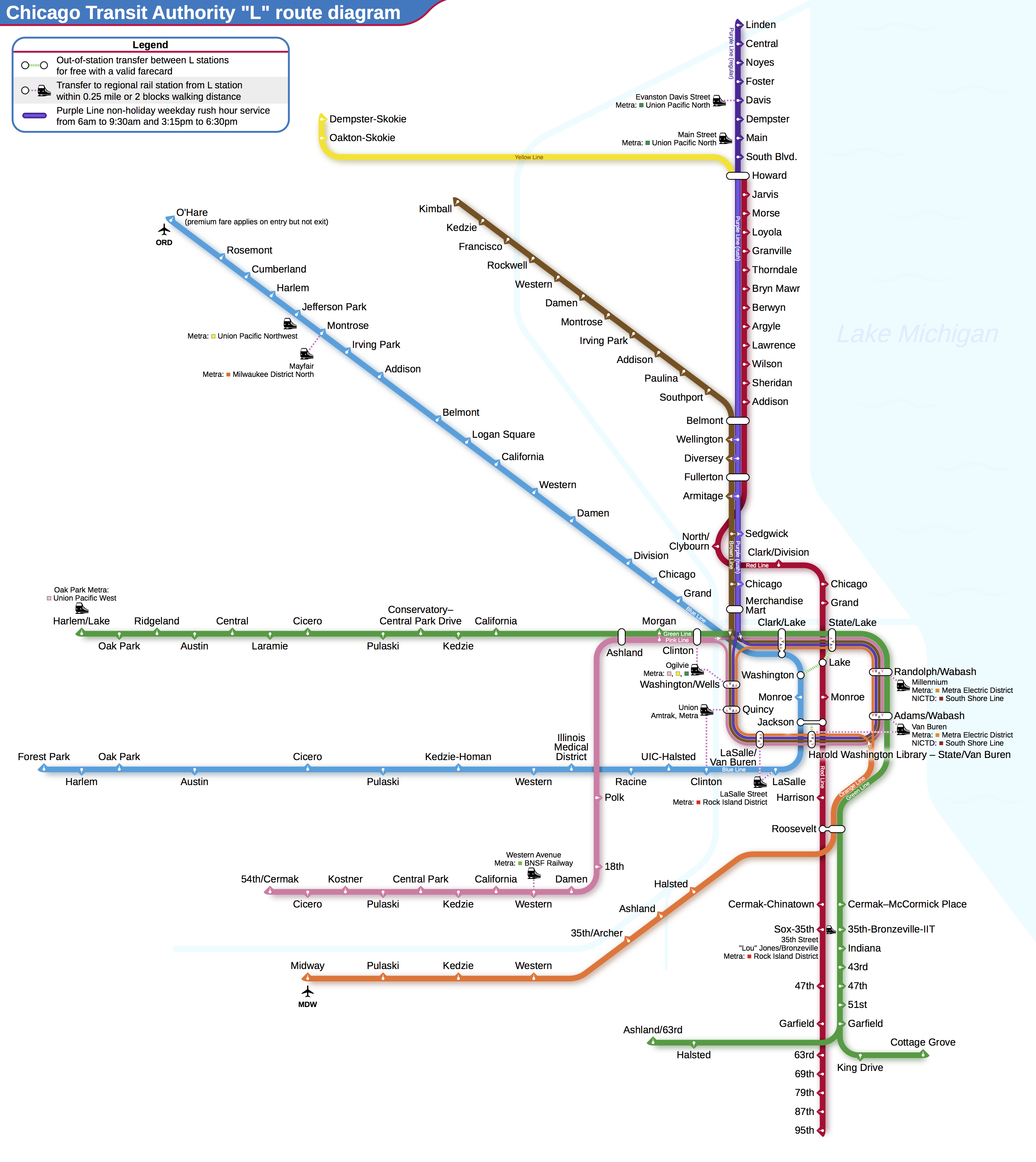Source: Wikimedia Commons, Creative Commons license

Since for any prediction of future ridership volume only historical values are available lagging data are used. In this case a lag of 14 days are used, i.e. ridership at day D-14.

Distribution of response

The distribution of the response gives an indication what to expect from a model. The residuals of a model should have less variation than the variation of the response.

If the distribution shows that the frequency of response decreases proportionally with larger values this might be an indication that the response follows a log-normal distribution. Log-transforming the response would induce a normal distribution and often will enable a model to have better prediction performance.

• Why look at distribution?• Gives indication on what to be expected from model performance
• variance of residuals < variance of response
• Distribution shaping might enable better prediction performance

A box plot gives a quick idea of the distribution of a variableFigure from

Box plot legend:

• Vertical line
• median of data
• Blue area
• represents 50% of data
• Whiskers
• indicate upper and lower 25% of data

Skewness of distribution

In the following picture the relative position of the red line within its surrounding box shows the skewness of the data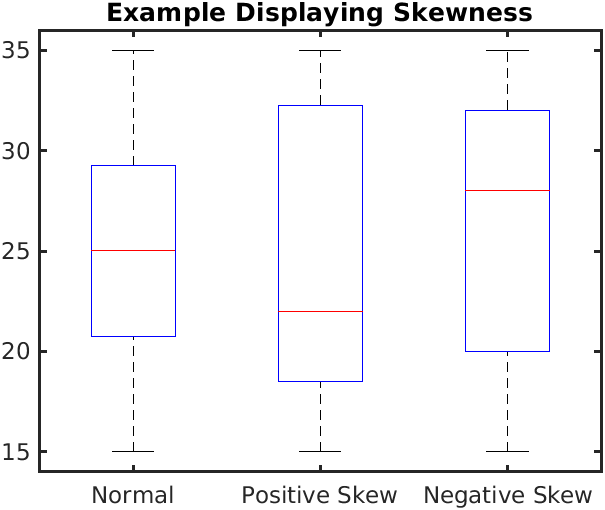Figure from Ever.chae [CC BY-SA]

What the skewness of data means for its distribution is shown in the picture belowFigure from Diva Jain [CC BY-SA]

mode: Value which appears most often in data set

The box plot doesn’t show if there are multiple peaks or modes. Histograms and violin plots are better suited in that caseFigure from

Box plot alternatives:

• Histogram
• data binned into equal regions
• height of bar proportional frequency of percentage of samples in region
• Violin plot
• compact visualization of distribution
• histogram-like characteristics
• lower quartile16
• median
• upper quartile17

To compare multiple distributions box plots are still helpful as shown in the next image which shows the distribution of weekday ridership at all stations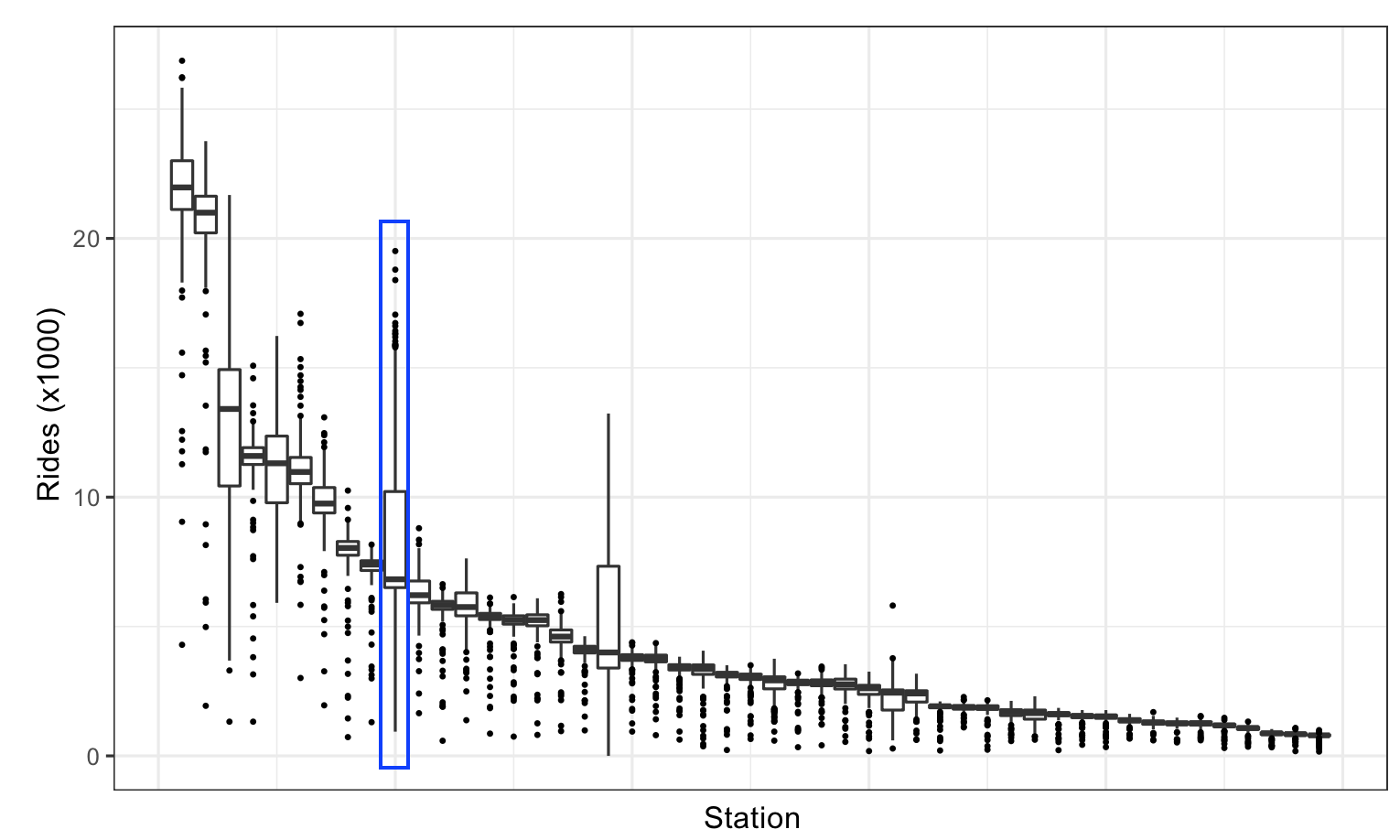Figure from

Knowledge gained through box plot:

• Wider distribution than other stations
• Station is close to stadium of Chicago Clubs
• \(\implies\) Clubs home game schedule would be important information for model

Using faceting and colors to augment visualizations

Facets create the same type of plots and splitting the plot into different panels based on some variable

Faceting:• Same type of plot
• Based on some variable
• Below faceting shows that ridership is different for parts of the week
• \(\implies\) part of the week is important feature

The plot below shows the ridership for Clark/Lake, and gives an explanation for the two modes seen in the histogram above, the ridership is vastly different on weekends than during the week.Figure from

Scatter plots

Scatter plots can add a new dimesions to the analysis

Scatter plot:

• One variable on x-axis, the other variable on y-axis
• Each sample plotted in this coordinate space
• Assess relationships
• between predictors
• between response and predictorsFigure from

There are several conclusions which can be drawn from the scatter plot above

Knowledge gained through scatter plot:

• Strong linear relationship between 14-day lag and current-day ridership
• Two distinct groups of points
• weekday
• weekend
• Plenty of outlier
• Uncovering explanation of outlier \(\implies\) new useful feature

Heatmaps

Heatmaps are a versatile plots that displays one predictor on the x-axis and another predictor on the y-axis. Both predictors must be able to be categorized. The categorized predictors form a grid, this grid is filled by another variable.

Heatmaps: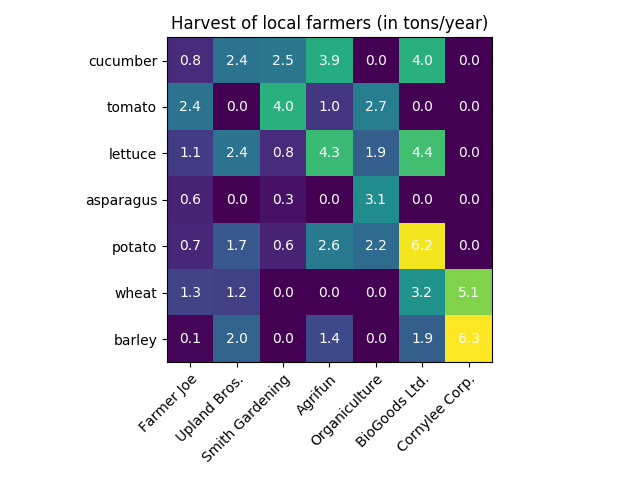• Categorize predictor
• for x and y-axis
• Display another variable on grid
• categorical or continuous
• Color depends on either value or category

The following heatmap investigates the all cases of weekday ridership less than 10,000. Those represent outlier needing explanation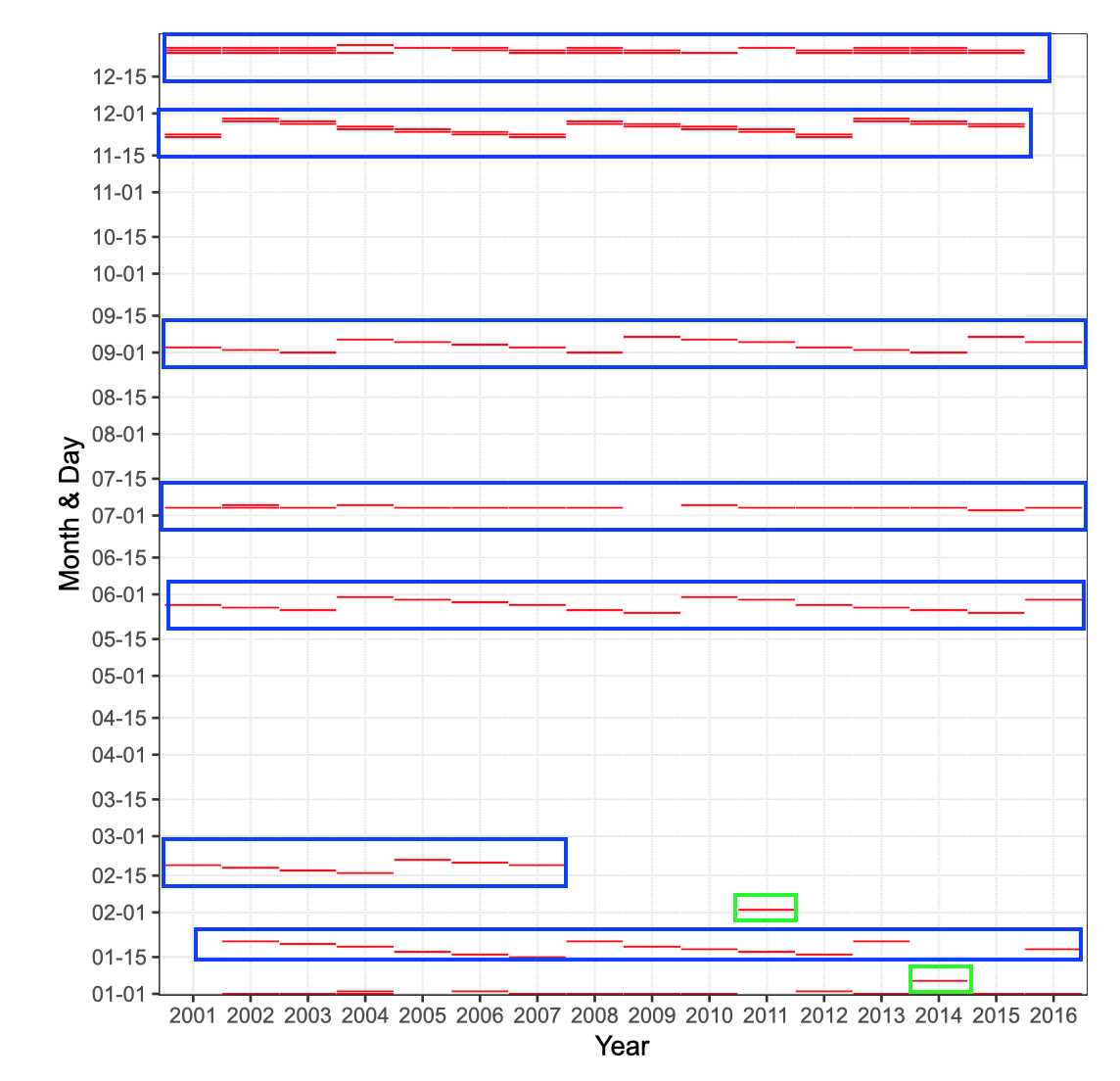Figure from

blue: holiday
green: extreme weather

Heatmap concept

• Categorize predictor
• x-axis: represents year
• y-axis: represents month and day
• Red lines indicate weekdays ridership < 10,000
• Blue boxes mark holiday seasons
• Green boxes mark unusual data points
• both days hat extreme weather
• \(\implies\) weather is important feature

Correlation matrix plots

An extension to scatter plot correlation matrix plots show the correlation between each pair of variable.

Correlation matrix plots

• Extension to scatter plot
• Each variable is represented on the outer x-axis and outer y-axis
• Matrix colored based on correlation value